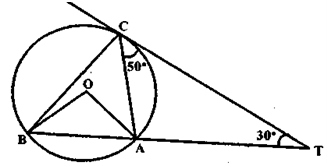In the figure below (not drawn to scale). A,B and C are there points on a circle with centre O. The chord BA is extended to a point T such that CT becomes a tangent to the circle at point C. if angle ATC = 30° and ACT = 50°, then the angle BOA is